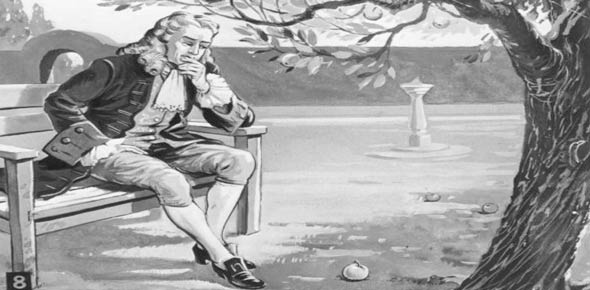# Newton's 2nd And 3rd Laws

7 Questions | Attempts: 278
ShareSettingsRemember F = ma and F1 = F2 (action = reaction)

• 1.
Suppose a cart is being moved by a certain net force. If the net force is doubled, by how much does its acceleration change?
• A.

Acceleration stays the same.

• B.

Acceleration is reduced by half.

• C.

Acceleration doubles.

• D.

Acceleration is squared.

• 2.
Suppose a cart is being moved by a certain net force. If a load is dumped into the cart so its mass is doubled, by how much does its acceleration change?
• A.

Acceleration stays the same.

• B.

Acceleration is reduced by half.

• C.

Acceleration doubles.

• D.

Acceleration is squared.

• 3.
Which produces more pressure on the ground: a person standing up or a person lying down?
• A.

A person standing up

• B.

A person lying down

• C.

They are equal

• D.

It depends on the time of day

• 4.
Which weighs more: a person standing up or a person lying down?
• A.

A person standing up

• B.

A person lying down

• C.

They are equal

• D.

It depends on the time of day

• 5.
A 1.0 kilogram ball accelerates at a rate of -24 meters per second squared when it hits a 5.0 kilogram table. Calculate the acceleration of the table. (Write your answer with the unit m/ss.)
• 6.
In example # 5, what force does the ball exert upon the table?
• 7.
In example #5, what force does the table exert upon the ball?

## Related TopicsBack to top
×

Wait!
Here's an interesting quiz for you.